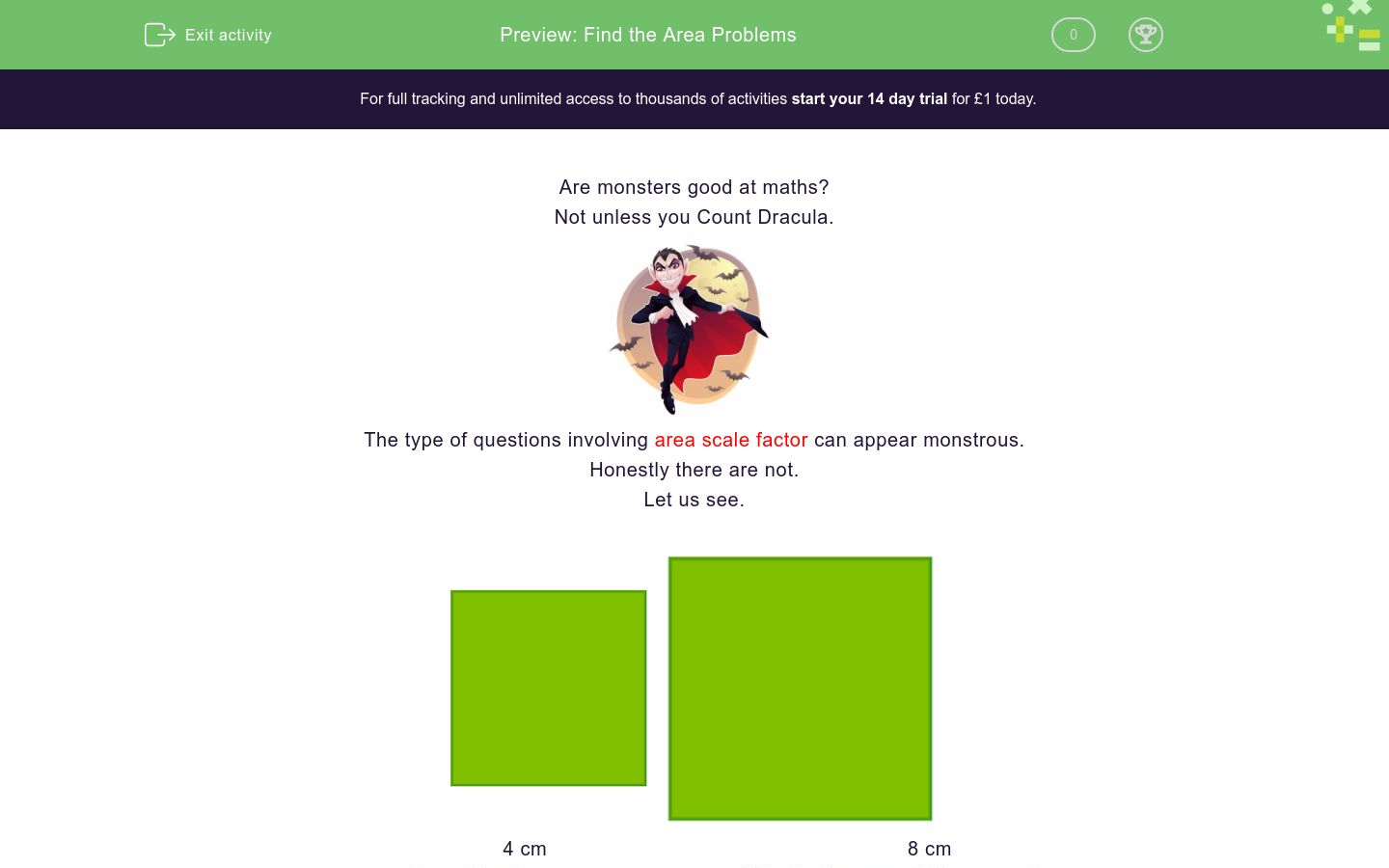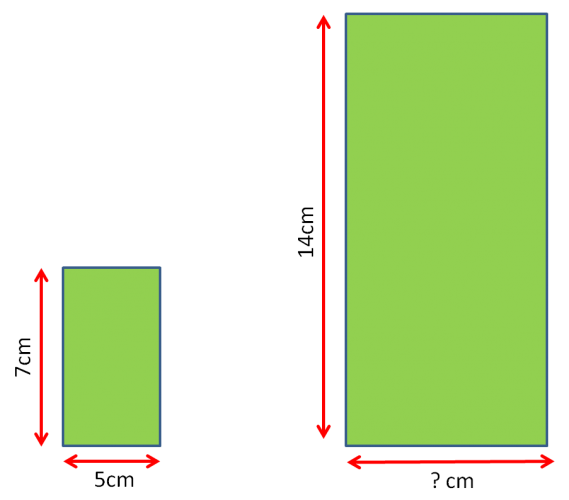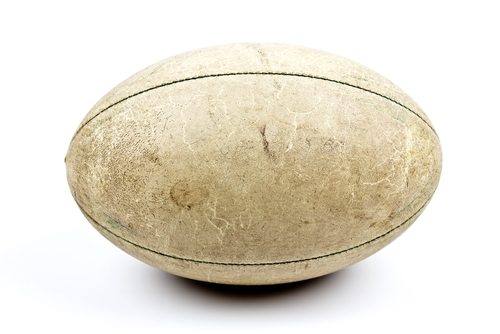# Find the Area Problems

In this worksheet, students will find the area of similar shapes.Key stage:  KS 4

GCSE Subjects:   Maths

GCSE Boards:   AQA, Eduqas, Pearson Edexcel, OCR

Curriculum topic:   Geometry and Measures, Congruence and Similarity

Curriculum subtopic:   Mensuration and Calculation, Similarity

Difficulty level:### QUESTION 1 of 10

Are monsters good at maths?

Not unless you Count Dracula.The type of questions involving area scale factor can appear monstrous.

Honestly there are not.

Let us see.4 cm                                                            8 cm

Area 16 cm²                                     What is the area of this square?

1.  Find the scale factor of the enlargement    8 ÷ 4  =  Scale factor 2

2.  THIS DOES NOT MEAN THAT THE AREA IS TWICE AS BIG.

3.  Take the scale factor and square it 2² = 4

4. The area of the larger square is 4 times as big

5. 16 x 4 = 64 cm²6  cm                                                      18 cm

Area = 12 cm²                                        What is the area of this rectangle?

1.  Find the scale factor of the enlargement    18 ÷ 6  =  Scale factor 3

2.  THIS DOES NOT MEAN THAT THE AREA IS THREE TIMES AS BIG.

3.  Take the scale factor and square it 3² = 9

4. The area of the larger rectangle is 9 times as big

5. 12 x 9 = 108 cm²

Let us put what we know already into a table

 Scale factor 2 Area scale factor 2² 4 times as big Scale factor 3 Area Scale factor 3² 9 times as big Scale factor 4 Scale factor 5

Can you spot the pattern and complete the rest of the table?

 Scale factor 2 Area scale factor 2² 4 times as big Scale factor 3 Area Scale factor 3² 9 times as big Scale factor 4 Area scale factor 4² 16 times as big Scale factor 5 Area scale factor 5² 25 times as big

This doesn't appear so monstrous after all does it?

These two triangles are similarBase 3 cm

Area = 14 cm²Base 9 cm

Whats the area of this triangle?

36 cm²

126 cm²

108 cm²

96 cm²What is the area of the larger rectangle?

These rectangles are similar.  What is the area of the larger rectangle?Side length 6 cm       Area = 48 cm²Side length 24 cmWhat is the area of the smaller rectangle?

60 cm²

30 cm²

20 cm²

15cm²

These two parallelograms are similar.  What is the area of the larger parallelogram?Base 3 cm  Area 150 cm²Base 15 cm

 450 cm² 1350 cm² 900 cm² 3750 cm² Area of the larger parallelogram

These two triangles are mathematically similar.

Base of arrow 4 cmBase of arrow 20 cm

Area of this arrow 500 cm²

Calculate the area of the smaller arrow.

100 cm²

30 cm²

20 cm²

50 cm²

These two cubes are mathematically similar.

The side of the smaller cube is 4 cm and the side of the larger cube is 12 cm.

The surface area of the larger cube is 864 cm²

What is the  surface area of the smaller cube?This rugby ball has a length of 8 cm and a total surface area of 90 cm²

A similar rugby ball has a length of 16 cm.  What is its surface area?

These two trapeziums are similar.Base 7 cm                                              Base 28 cm

Area 40 cm²

Find the area of the larger trapezium.

 460 cm² 640 cm² 520 cm² Area of the larger trapeziumThese two cylinders are mathematically similar.  The height of the larger cylinder is twice the height of the smaller cylinder.

The total surface area of the smaller cylinder is 160 cm²

Calculate the total surface area of the larger cylinder.

Write you answer in figures without the units.

• Question 1

These two triangles are similarBase 3 cm

Area = 14 cm²Base 9 cm

Whats the area of this triangle?

126 cm²
EDDIE SAYS
First find the scale factor 9 ÷3 = 3 Find area scale factor 3² = 9 The area of the larger triangle is 9 times as big 9 x 14 = 126 cm² Why did 7 eat 9? Because you are supposed to eat three square meals a day.
• Question 2What is the area of the larger rectangle?

140
EDDIE SAYS
Are you scaling to new heights in your knowledge? Find the scale factor 14 ÷ 7 = 2 Area scale factor is 2² = 4 Area is 4 times as big. Smaller rectangle has an area of 7 x 5 = 35 cm² 35 x 4 = 140 cm² A little bit more to do here as you are not given the area but it doesn't take much to do that does it?
• Question 3

These rectangles are similar.  What is the area of the larger rectangle?Side length 6 cm       Area = 48 cm²Side length 24 cm

EDDIE SAYS
Scale factor 24 ÷ 6 = 4 Scale factor 4² = 16 The area is 16 times larger 48 x 16 = 768 cm² Who would have thought that square numbers would come in so useful.
• Question 4What is the area of the smaller rectangle?

15cm²
EDDIE SAYS
As long as you are familiar with square numbers this should become second nature to you. Scale factor 6 ÷ 2 = 2 Area Scale Factor = 2² = 4 times as small As it is smaller we just divide. Area of the larger triangle is 10 x 6 = 60 cm² 60 ÷ 4 = 15 cm²
• Question 5

These two parallelograms are similar.  What is the area of the larger parallelogram?Base 3 cm  Area 150 cm²Base 15 cm

 450 cm² 1350 cm² 900 cm² 3750 cm² Area of the larger parallelogram
EDDIE SAYS
Scale factor 15 ÷ 3 = 5 Area scale factor is 5² = 25 25 times bigger 150 x 25 = 3750 cm²
• Question 6

These two triangles are mathematically similar.

Base of arrow 4 cmBase of arrow 20 cm

Area of this arrow 500 cm²

Calculate the area of the smaller arrow.

20 cm²
EDDIE SAYS
Is everything now pointing in the right direction for you? Scale factor 20 ÷ 4 = 5 Area scale factor is 5² = 25 500 ÷ 25 = 20 cm² As long as you remember the area scale factor and not use the general scale factor you will always stay on the right track.
• Question 7

These two cubes are mathematically similar.

The side of the smaller cube is 4 cm and the side of the larger cube is 12 cm.

The surface area of the larger cube is 864 cm²

What is the  surface area of the smaller cube?96
EDDIE SAYS
Don't let the word surface area put you off, because the shape is now 3D. The same process applies because it is still area. Scale factor is 12 ÷ 4 = 3 Area Scale factor is 3² = 9 Smaller cube has a surface area 9 times smaller 864 ÷ 9 = 96 cm²
• Question 8This rugby ball has a length of 8 cm and a total surface area of 90 cm²

A similar rugby ball has a length of 16 cm.  What is its surface area?

EDDIE SAYS
Oh I do like a game of rugby, (watching not playing, that would hurt to much) As you can see you can use area scale factor for a variety of objects. Scale factor 2 Area scale factor 4 90 x 4 = 360 cm²
• Question 9

These two trapeziums are similar.Base 7 cm                                              Base 28 cm

Area 40 cm²

Find the area of the larger trapezium.

 460 cm² 640 cm² 520 cm² Area of the larger trapezium
EDDIE SAYS
28 ÷ 7 = 4 Area scale factor is 4² = 16 Larger trapezium is 16 times as big 40 x 16 = 640 cm²
• Question 10These two cylinders are mathematically similar.  The height of the larger cylinder is twice the height of the smaller cylinder.

The total surface area of the smaller cylinder is 160 cm²

Calculate the total surface area of the larger cylinder.

Write you answer in figures without the units.

620
EDDIE SAYS
It is the wording on this that you need to watch as there is no measurement for the height. Twice as big should tell you the scale factor is 2 therefore area scale factor is 2² which is 4 times bigger. 160 cm x 4 = 640 cm²
---- OR ----

Sign up for a £1 trial so you can track and measure your child's progress on this activity.

### What is EdPlace?

We're your National Curriculum aligned online education content provider helping each child succeed in English, maths and science from year 1 to GCSE. With an EdPlace account you’ll be able to track and measure progress, helping each child achieve their best. We build confidence and attainment by personalising each child’s learning at a level that suits them.

Get started